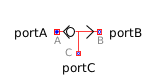Root Mean Square - MapleSim Help

Root Mean Square

Compute the root mean squareDescription The Root Mean Square component computes the root-mean-square of the input signal $u$ over the period of a specified frequency $f$.Equations $y=\left\{\begin{array}{cc}0& t<{t}_{0}+\frac{1}{f}\\ \sqrt{f{\int }_{T}^{T+\frac{1}{f}}{u}^{2}\mathrm{dt}}& \mathrm{otherwise}\end{array}$ $T={t}_{0}+\frac{\left|\left(t-{t}_{0}\right)f\right|-1}{f}$ $\mathrm{with}{t}_{0}\mathrm{the start-time of the simulation}$Connections

 Name Description Modelica ID $u$ Real input signal u $y$ Real output signal yParameters

 Name Default Units Description Modelica ID $f$ $50$ $\mathrm{Hz}$ Base frequency fModelica Standard Library The component described in this topic is from the Modelica Standard Library. To view the original documentation, which includes author and copyright information, click here.